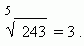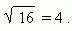# Arithmetical operations

Multiplication (multiplicand,  multiplier,  product,  factors). Division
(dividend, divisor, quotient, dividing integers, fraction, divisible numbers,
remainder, division without remainder, division with remainder). Raising
to a power
(power, base of a power, index or exponent of a power, value
of a power). Extraction of a root (root, radicand, index or degree of a
root, value of a root, square root, cube root). Mutually inverse operations.

Addition – an operation of finding a sum of some numbers: 11 + 6 = 17. Here 11 and 6 – addends ,  17 – the sum . If addends are changed by places, a sum is saved the same: 11 + 6 = 17 and 6 + 11 = 17.

Subtraction – an operation of finding an addend by a sum and another addend: 17 – 6 = 11.  Here 17 is a minuend ,  6 – a subtrahend ,  11 – the difference .

Multiplication. To multiply one number n ( a multiplicand ) by another m ( a multiplier ) means to repeat a multiplicand n as an addend m times. The result of  multiplying is called a product. The operation of multiplication is written as: n x m or n · m . For example, 12 x 4 = 12 + 12 + 12 + 12 = 48. In our case 12 x 4 = 48 or 12 · 4 = 48. Here 12 is a multiplicand, 4 – a multiplier, 48 – a product. If a multiplicand n and a multiplier m are changed by places, their product is saved the same:  12 · 4 = 12 + 12 + 12 + 12 = 48 and 4 ·12 = 4 + 4 + 4 + 4 + 4 + 4 + 4 + 4 + 4 + 4 + 4 + 4 = 48. Therefore, a multiplicand and a multiplier are called usually factors or multipliers.

Division – an operation of finding one of factors by a product and another factor: 48 : 4 = 12. Here  48  is a dividend ,  4 – a divisor ,  12 – the quotient . At dividing integers a quotient can be not a whole number. Then this quotient can be present as a fraction . If a quotient is a whole number, then it is called that numbers are divisible , i.e. one number is divided without remainder by another. Otherwise, we have a division with remainder . For example, 23 isn’t divided by 4 ; this case can be written as:  23 = 5 · 4 + 3.  Here 3 is a remainder .

Raising to a power. To raise a number to a whole (second, third, forth, fifth etc.) power means to repeat it as a factor two, three, four, five and so on. The number, repeated as a factor, is called a base of a power ; the quantity of factors is called an index or an exponent of a power ; the result is called a value of a power . A raising to a power is written as:

3 5 = 3 · 3 · 3 · 3 · 3 = 243 .

Here  3 – a base of the power,  5 – an exponent (an index) of the power,  243 – a value of the power.
The second power is called a square , the third one – a cube . The first power of any number is this number.

Extraction of a root – an operation of finding a base of a power by the power and its exponent:Here 243 – a radicand , 5 – an index ( degree ) of the root , 3 – a value of the root . The second root is called a square root, the third root – a cube root .The second degree of square root isn’t written:Addition and subtraction, multiplication and division, raising to a power and extraction of a root are two by two mutually inverse operations .